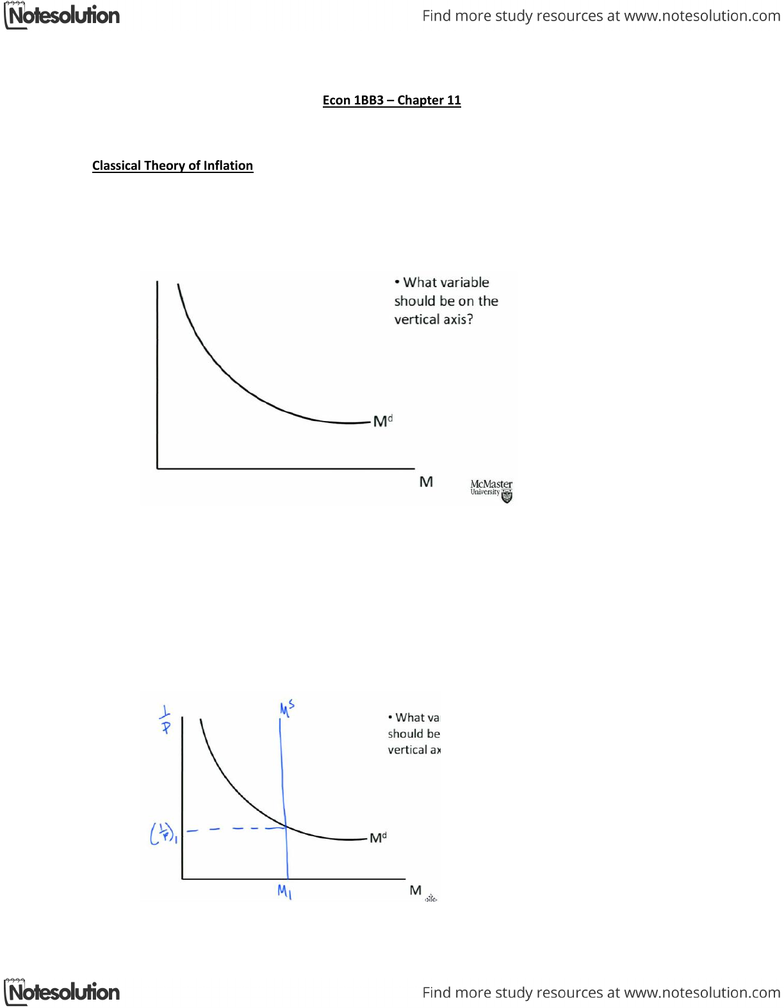# ECON 1BB3 Lecture Notes - Classical Dichotomy, Money Supply, Hot Chocolate

30 views3 pages
School
McMaster University
Department
Economics
Course
ECON 1BB3
Page:
of 3Richard Damra Tuesday, February 26, 2013
Econ 1BB3 Chapter 11
Why is the inflation rate 2.5% in Canada but 19% in Venezuela?
Classical Theory of Inflation
Price of a good reflects value
Money Value falls as the price level rises
Demand for money (households) as prices rise we need more money to buy the same goods.
The demand for money curve has a negative slope
When P goes up, value of money goes down
Vertical axis = 1/P (Value of money)
If 1/P goes down P goes up and vice versa
Md (Money Demand) shift out if Y rises or if households decide to increase their money
holdings for some other reason.
Y stands for several things, depends on context, in this context, Income. Can also be GDP
(Output).
Ms (Money Supply) vertical position determined by the central bank
Increasing the money supply shifting the line to the right, decreasing shifting to the left.
Richard Damra Tuesday, February 26, 2013
Quantity theory of Money
Quantity theory of money:
o The quantity of money determines the price level
o The growth rate of money determines the inflation rate
o The faster the money is printed the faster the growth of inflation rate (Answer to
lecture’s question
Classical dichotomy: the separation of real and nominal variables. Real variable are measured in
physical quantities or their relatives’ i.e sack of potatoes in terms of rice or measured in some
form of constant dollars. Nominal is measured in terms of current dollars. Real variables are
determined by other real variables
Monetary neutrality: Changes in Ms affect nominal variables, but not real variables.
Velocity
Velocity: rate at which money changes hands
Example: produce 2000 cups of hot chocolate / week
o \$2 per cup
o \$500 of currency in the economy
o How many times must each dollar circulate through the economy for all the hot
chocolate to be purchased?
o 8 times
Quantity Equation
The growth rate of the product of two variables is (approximately) equal to the sum of the
growth rates of the individual variables.
Richard Damra Tuesday, February 26, 2013
Question 1 from Page 264
Suppose that this year’s money supply is \$50 billion, nominal GDP is \$1 trillion, and real GDP is
\$500 billion.
o A) What is price level? What is velocity of money?
Py = \$1000 Billion M=\$50 Billion y=\$500billion
P= Py/y = 1000/500 = 2 Price level
MxV=PxY
V=PxY/M=1000/50=20 Velocity
o B) Suppose that velocity is constant and the economy’s output of goods and services
rises by 5 percent each year. What will happen to nominal GDP and the price level next
year if the Bank of Canada keeps the money supply constant.
Nominal GDP is constant because Velocity is constant which makes M constant
and makes the right side of the equation MxV = PxY constant
o C) What money supply should the bank of Canada set next year if it wants to keep the
price level stable
Increase M by 5% therefore M=\$52.5 billion
o D) What money supply should the bank of Canada set next year if it wants inflation of
10%?
P increase by 10% and Y increase by 5%
Money supply should increase by 15% therefore M = \$57.5B

## Document Summary

Price of a good reflects value. Money value falls as the price level rises. Demand for money (households) as prices rise we need more money to buy the same goods. The demand for money curve has a negative slope. When p goes up, value of money goes down. Vertical axis = 1/p (value of money) Md (money demand) shift out if y rises or if households decide to increase their money. If 1/p goes down p goes up and vice versa holdings for some other reason. Y stands for several things, depends on context, in this context, income. Ms (money supply) vertical position determined by the central bank. Increasing the money supply shifting the line to the right, decreasing shifting to the left. Classical dichotomy: the separation of real and nominal variables. Real variable are measured in physical quantities or their relatives" i. e sack of potatoes in terms of rice or measured in some form of constant dollars.